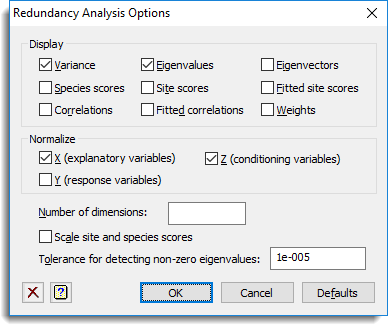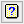1. Home
2. Redundancy Analysis Options

# Redundancy Analysis Options

Use this to select options and output to be used by redundancy analysis.## Display

Specifies which items of output are to be displayed in the Output window.

 Variance The fraction of the variance of the response variates associated with each eigenvalue Eigenvalues The eigenvalues of the fitted values (also known as the species scores) Eigenvectors The eigenvectors associated with each eigenvalue Species scores The species scores from the analysis Site scores The site scores of the response variables (i.e. the ordination of the units in the response variable (Y) space Fitted site scores The fitted site scores of the fitted values of the response variables (i.e. the ordination of the units in the response variable space) Correlations The correlation between the site scores and the explanatory variables Fitted correlations The correlation between the fitted site scores and the explanatory variables Weights The weights of the explanatory variables in the formation of the site scores

## Normalize

Specifies whether the data should be normalized to have unit sums-of-squares before analysis.

 X (explanatory variables) Normalizes the explanatory variables (X-variates) Y (response variables) Normalizes the response variables (Y-variates) Z (conditioning variables) Normalizes the conditioning variables (Z-variates)

## Number of dimensions

Specifies the number of eigenvalues and eigenvectors to include in output. If this is left blank then all eigenvalues and eigenvectors will be displayed.

## Scale site and species scores

Controls scaling for species and site scores. When selected, both species and site scores are multiplied by the square root of their corresponding eigenvalues.

## Tolerance for detecting non-zero eigenvalues

Specifies a threshold for the detection of non-zero eigenvalues. An eigenvalue is taken to be non-zero if is it greater than tolerance multiplied by the total variance.

## Action IconsClear Clear all fields and list boxes.Help Open the Help topic for this dialog.